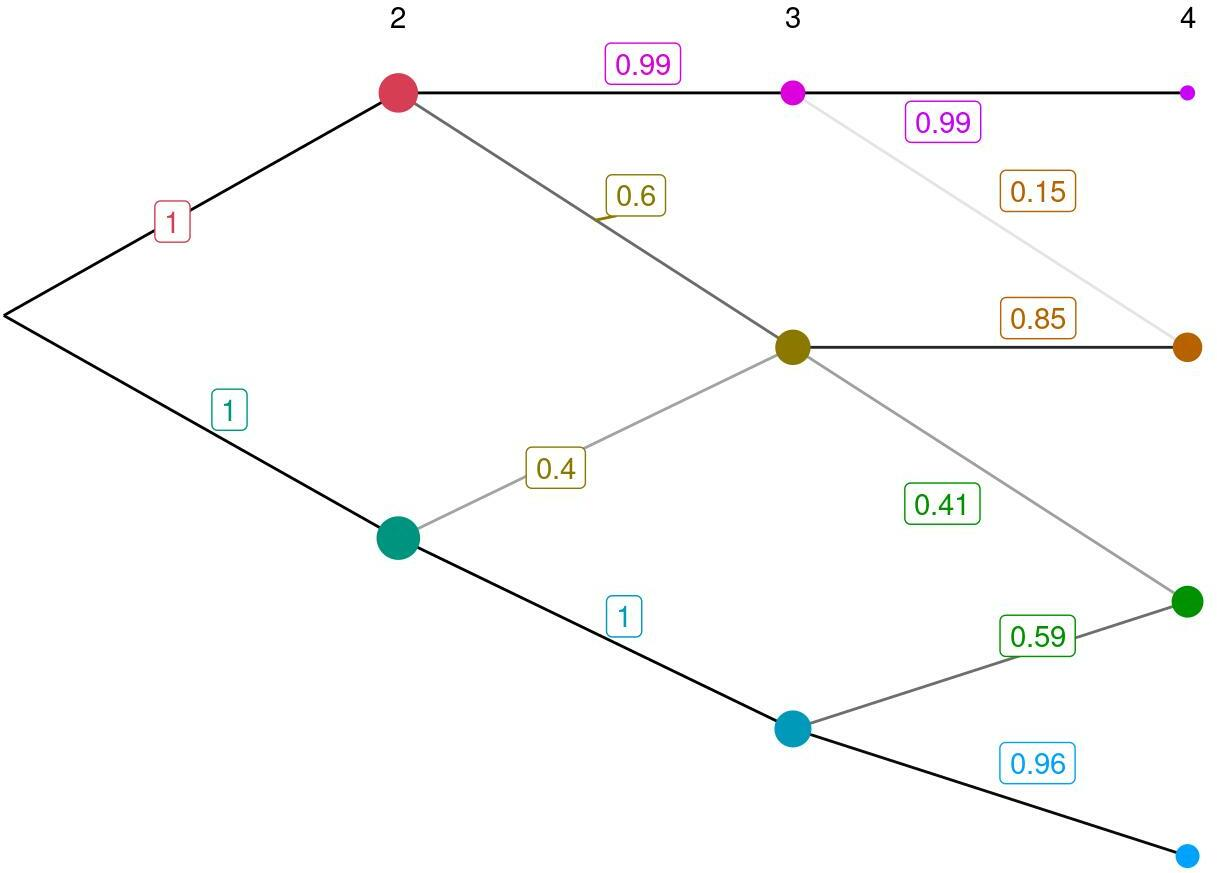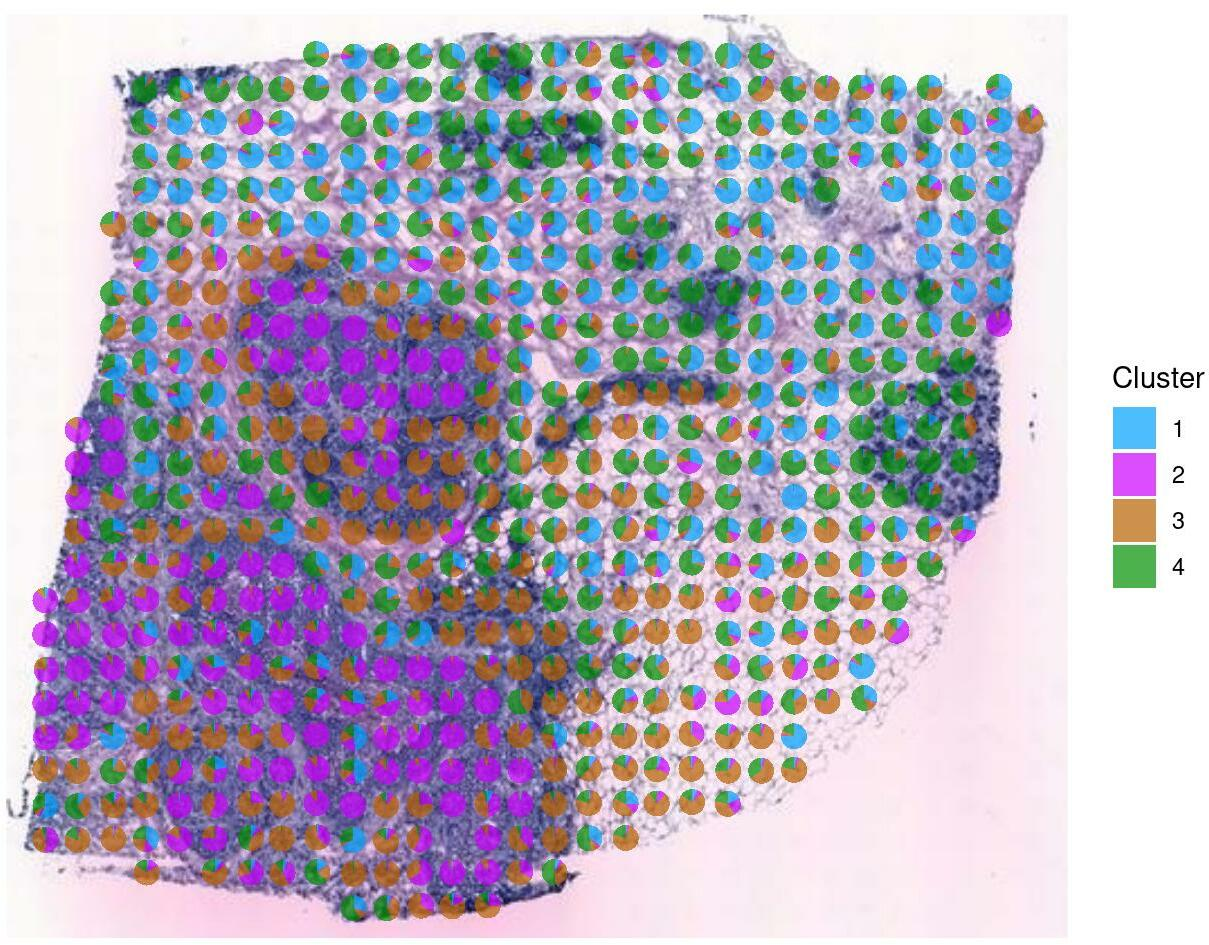# 1 Notice to users using OSX with R-devel

SpatialCPie depends on the ggiraph package, for which their is no r-devel version available at the moment. This means that SpatialCPie will not work on OSX with R-devel.

# 2 Introduction

SpatialCPie is an R package designed to facilitate cluster evaluation for Spatial Transcriptomics (ST) data by providing intuitive visualizations that display the relationship between clusters in order to guide the user during cluster identification, selection and further downstream applications

# 3 Usage example

library(SpatialCPie)
set.seed(42)

## 3.1 Input data

In this example, we will use a downsampled dataset from an experiment on the human heart. We begin by loading the count data1 Generated by the ST pipeline:

counts <- read.table(
system.file("extdata", "counts.tsv", package = "SpatialCPie"),
sep = "\t",
check.names = FALSE
)
counts[1:5, 1:5]
# >        3x34  3x30  3x31  3x32  3x33
# > ACTB  2.511 2.116 2.910 3.792 2.432
# > CD74  1.744 2.323 1.666 3.061 0.000
# > CFL1  0.000 2.116 1.666 1.909 2.432
# > CST3  3.009 2.664 0.000 3.061 2.925
# > ERBB2 1.744 3.461 0.000 3.473 3.584

To overlay the data on the tissue, we also need to load the tissue image and its corresponding spot data2 Generated by the ST spot detector., which specifies the pixel coordinates of each spot:

tissue <- jpeg::readJPEG(
system.file("extdata", "he_image.jpg", package = "SpatialCPie")
)
spots <- parseSpotFile(
system.file("extdata", "spot_data.tsv", package = "SpatialCPie")
)
# >             x        y
# > 11x9 149.3096 119.4788
# > 12x9 166.1704 120.5682
# > 13x9 180.5411 120.4645
# > 14x9 195.2749 120.3607
# > 15x9 209.6456 120.3607
# > 16x9 225.3132 120.9314

## 3.2 Preprocessing

Typically, it’s good to conduct some data filtering prior to the analysis: This could include removing spots that are outside of the tissue or removing spots or genes that have a low number of reads.

Since the spot file only contains the spots that are under the tissue, we can use it to subset the counts:

counts <- counts[, which(colnames(counts) %in% rownames(spots))]

Let’s also remove all spots and genes that have less than 20 reads in total:

repeat {
d <- dim(counts)
counts <- counts[rowSums(counts) >= 20, colSums(counts) >= 20]
if (all(dim(counts) == d)) {
break
}
}

Our data has already been normalized. If you are using raw count data, it is usually a good idea to normalize it before proceeding. We have found SCTransform in the Seurat package to work well for Spatial Transcriptomics data.

## 3.3 Computing cluster assignments

The cluster assignments will be calculated during the loading of the gadget. The cluster assignments are labels assigned to each spot over different cluster resolutions, where we use the terminology “(cluster) resolution k” to refer to a partitioning of the spots into $$k$$ clusters.

By default, clustering is performed with base::kmeans at resolutions 2:4. The arguments resolutions and assignmentFunction to runCPie can be used to customize these defaults. For example, to instead cluster over resolutions 3 and 5 with cluster::pam, call runCPie as follows:

runCPie(
counts,
resolutions = c(3, 5),
assignmentFunction = function(k, x) cluster::pam(x, k = k)$clustering ) Use ?runCPie for a complete list of function options. ## 3.4 Visualization We are now ready to use the gadget to visualize the data: result <- runCPie( counts, image = tissue, spotCoordinates = spots ) # > Clustering resolution 2L # > Clustering resolution 3L # > Clustering resolution 4L # > Maximizing label overlap in consecutive resolutions # > Scoring spot-cluster affinity This opens up the gadget window, which has two main elements: the cluster graph and the spatial array plots. The cluster plot is interactive and by selecting rows of nodes, the corresponding spatial array plots are also displayed. These can be viewed by scrolling down. The information contained in these plots will be discussed in the next section. There are a number of parameters that can be changed within the gadget which affects the visual representation. To exit the gadget, press the “Done” button. The plots in the gadget and the cluster assignments are stored in the output object, which has the following structure: str(result, max.level = 1) # > List of 3 # >$ clusters    :'data.frame':   2400 obs. of  3 variables:
# >  $clusterGraph:List of 10 # > ..- attr(*, "class")= chr [1:2] "gg" "ggplot" # >$ arrayPlot   :List of 4

result$clusterGraphThe cluster graph is a visual representation of how the spatial features transition from clusters of lower resolution to clusters of higher resolution. The edge opacities show the proportion of spots that transition to or from a given cluster while node radii display the size of each cluster. When launching the gadget, spots are relabeled so as to minimize the number of crossovers (label switches) between resolutions in the data. Moreover, cluster color labels are selected so that dissimilar clusters are farther away in color space. ### 3.4.2 Spatial array plots result$arrayPlot\$4The spatial array plots show the spatial locations of the clusters. The pie chart proportions are based on the following scoring scheme.

#### 3.4.2.1 Spot-based clustering

In the case of spot-based clustering (margin = "spot" in the call to runCPie, which is the default), the score for cluster $$k$$ in spot $$s$$ is computed as:

$\mbox{score}(s, k) = \mbox{exp}\left( - \mbox{RMSD}\left(x_s, \mbox{mean}({\{x_{s'}\}_{s' \in C(k)}})\right) \right),$

where $$x_i$$ is the gene expression vector of spot $$i$$, $$C(k)$$ is the set of spots in cluster $$k$$, and $$\mbox{RMSD}(a, b)$$ is the root-mean-square deviation between gene vectors $$a$$ and $$b$$.

#### 3.4.2.2 Gene-based clustering

In the case of gene-based clustering (margin = "gene" in the call to runCPie), the score is computed as:

$\mbox{score}(s, k) = \mbox{mean}\left( {\{\tilde{x}_{sg}\}_{g \in C(k)}} \right),$

where $$\tilde{x}_{sg}$$ is a log-normalized relative count value for gene $$g$$ in spot $$s$$.

#### 3.4.2.3 The score multiplier

The score can be tuned by a score multiplier, $$\lambda$$, to either enhance or decrease the contrast between clusters. The final (visualized) score is an exponentiation of $$\mbox{score}$$:

$\mbox{score'}(s, k) = \mbox{score}(s, k) ^ \lambda$

Note that the definition implies that it is possible to (practically) hard-label the spots by setting $$\lambda$$ to a high value. This follows since the relative score for the highest scoring cluster will tend towards $$1$$ as $$\lambda\to\infty$$. For example, setting $$\lambda=10.0$$ yields